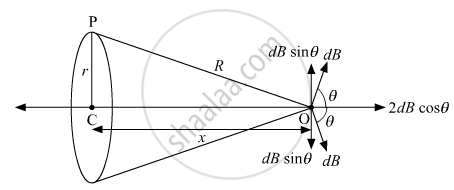# Derive the expression for the magnetic field due to a current-carrying coil of radius r at a distance x from the center along the X-axis. - Physics

Numerical

Derive the expression for the magnetic field due to a current-carrying coil of radius r at a distance x from the center along the X-axis.

#### SolutionConsider a conducting element dl of the loop. The magnitude dB of the magnetic field due to dl is given by the Biot-Savart law,

"dB" = mu_°/(4pi) ("i"|vec(dl) xx vec("R")|)/"R"^3

"dB" = mu_° /(4pi) ("idl")/"R"^2 (vec"idl" ⊥ vec"R")

From the above figure, we can see, R2 = r2 +x2

"dB" = mu_° /(4pi) xx "idl"/("r"^2 + "x"^2)

The direction of the magnetic field is shown in the figure, as we can see, only cosine component of the magnetic field will play a role here, all the sine component will get cancel out to give a zero net value.

"dB"_"net" = mu_°/(4pi) xx "idl"/(("r"^2 + "x"^2)) xx costheta

"dB"_"net" = mu_°/(4pi) xx "idl"/(("r"^2 + "x"^2)) xx "r"/(sqrt("r"^2+"x"^2))

"dB"_"net" = (mu_° "idl")/(4pi) "r"/(("r"^2 + "x"^2)^(3//2))

∵ dl = rdθ

⇒ "B"_"net" = (mu_°"i")/(4pi) ("r"^2 int_0^(2pi) "d"theta)/(("r"^2 + "x"^2)^(3//2)) = (mu_°"ir"^2)/(2("r"^2+ "x"^2)^(3//2))

⇒ "B"_"net" = (mu_°"ir"^2)/(2("r"^2+ "x"^2)^(3//2))

⇒ vec("B"_"net") = (mu_°"ir"^2)/(2("r"^2+ "x"^2)^(3//2)) hat"i"

Concept: Magnetic Field Due to a Current Element, Biot-Savart Law
Is there an error in this question or solution?